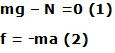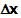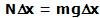## Thursday, February 12, 2009

### Irodov Problem 1.271There are three forces acting on the cube during its motion, i) the force of gravity mg acting downwards, ii) the normal reaction N from the surface acting upwards and iii) the force of friction f opposing the motion of the cube. Let the acceleration of the cube in the horizontal direction be a. Then we have,The maximum possible force of friction f offered by the surface is given by Nk. Since the cube is moving, the maximum force of friction is acting on the cube. In other words, f = Nk. So, from (2) we have,From (1) and (3) it is now clear that,In (5), vo is the initial velocity of the cube.

Now let us consider the torques acting on the cube about and axis AA' that passes through the cube CG, is perpendicular to the velocity vector and lies in a plane parallel to the surface. The force of gravity mg acts through the CG (along the line OO') and hence does not induce any torque about AA'. The force of friction acts at the surface uniformly at all points of contact and hence acts at a distance a/2 from AA' and induces a torque of mgka/2. This must mean that the force friction should have a tendency to rotate the cube in the clockwise direction about the CG. However, the cube does not rotate. This is because the normal reaction N, shifts away from the axis passing through the CG (OO') to another parallel axis BB' and induces a counter-torque to that induced by the force of friction and exactly cancels it.

Suppose that the axis BB' is at a distancefrom the axis OO'. Then, the torque induced by the normal reaction about the axis AA' is given by. Hence, for the cube not to rotate about the axis AA' we have,Now let us consider the question of dissapearance of angualr momentum about an axis CC' that lies in the surface and is perpendicular to the direction of motion of the cube.Suppose at some instant of time, the CG of the cube is at a distance x from the axis CC'. The force of friction passes through this axis and hence induces no torque whatsoever. There are however, two other torques acting on the cube i) that due to gravity given by mgx and ii) that due to the normal reaction -N(x+ak/2) = -mg(x+ak/2).

Thus, there is a net torque of -mgak/2 acting on the cube at any given point of time w.r.t to the axis CC'. The initial angular momentum of the cube w.r.t to the axis CC' is mvoa/2 is thus, reduced as time passes given by,This clearly explains how angular momentum is lost w.r.t to the axis CC'.